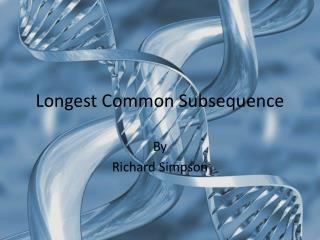Download PresentationLongest Common Subsequence# Longest Common Subsequence - PowerPoint PPT Presentation

Download Presentation##### Longest Common Subsequence

Download Policy: Content on the Website is provided to you AS IS for your information and personal use and may not be sold / licensed / shared on other websites without getting consent from its author. While downloading, if for some reason you are not able to download a presentation, the publisher may have deleted the file from their server.

- - - - - - - - - - - - - - - - - - - - - - - - - - - E N D - - - - - - - - - - - - - - - - - - - - - - - - - - -
##### Presentation Transcript

1. Longest Common Subsequence By Richard Simpson

2. Subsequence of a string • A subsequenceof a sequence(or string) X is and sequence obtained from X by removing 0 or more of the characters of X. The remaining characters are kept in the same order. • Example X=“abfrdfdvredffgfd” Removing the red characters gives us “abrdfredfffd” which is a subsequence of X.

3. Common Subsequence • A common subsequence of sequences X and Y is a just a string that is a subsequence of both X and Y. • There may be many subsequences of X and Y. A subsequence of this set that has maximal length is called the longest common subsequence. • There may be several of these maximal subsequences.

4. How many subsequences are there for a subseq of length n? • This is related to the number of subsets of a set. How many are there? • 2 to the n • Why? • Every time you add a new character you double the number of subsets. Do it by hand and check it out. • SO! Brute force is out!!!

5. ith Prefix • Let X be a sequence • Then Xi is defined as the first i characters of the sequence X.d • For example let X= “asdcfrfgtr” thenX4 is the sequence “asdc” • We will use these prefix's to build the LCS

6. Optimal Substructure of an LCS Let C = x1x2x3…xm and Y = y1y2y3…yn and let Z= z1z2z3…zk be any LCS of X and Y. • If xm=yn then zk=xm=yn and Zk-1 is and LCS of Xm-1 and Yn-1 • If xm≠ynthen zk ≠ xm implies that Z is an LCS of Xm-1 and Y • If xm≠ynthen zk ≠ ymimplies that Z is an LCS of X and Yn-1

7. Meaning? • If xm=yn then zk=xm=yn and Zk-1 is and LCS of Xm-1 and Yn-1 X = acdggdcagagccda Y = acdgadcggagdada Z = acdgdcgagda This is similar for the 3rd case

8. Meaning? • If xm≠ynthen zk ≠ xmimplies that Z is an LCS of Xm-1 and Y X = acdggdcagagccdc Y = acdgadcggagdada Z = acdgdcgagda

9. Recursive View Let c[i,j] be the length of the LCS of prefixes Xi and Yjthe the optimal substructure of the LCS problem gives us

10. The Algorithm # Initialize the arrays m=length[X]; n=length[Y] For i=1 to n do c[i,0]=0 For j=1 to n do c[0,j]=0

11. Continued For i=1 to m do for j=1 to n do if xi=yj then c[i,j] = c[i-1,j-1]+1 b[i,j] = “↖” else if c[i-1,j]>=c[i,j-1] then c[i,j]=c[i-1,j] b[i,j]=“↑” else c[i,j]= c[I,j-1] b[i,j]=“←” Return c and b

12. The Table

13. Print it Print-LCS(b,X,i,j) if i=0 or j=0 return if b[I,j]=“↖” then Pirnt-LCS(b,X,i-1,j-1) print xi elseif b[i,j]=“↑” then Print-LCS(b,X,i-1,j) else Print-LCS(b,X,i,j-1)